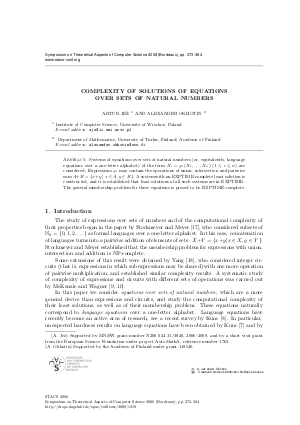Document# Complexity of solutions of equations over sets of natural numbers

### Authors Alexander Okhotin, Artur Jez## File

LIPIcs.STACS.2008.1319.pdf
• Filesize: 179 kB
• 12 pages

## Cite As

Alexander Okhotin and Artur Jez. Complexity of solutions of equations over sets of natural numbers. In 25th International Symposium on Theoretical Aspects of Computer Science. Leibniz International Proceedings in Informatics (LIPIcs), Volume 1, pp. 373-384, Schloss Dagstuhl - Leibniz-Zentrum für Informatik (2008)
https://doi.org/10.4230/LIPIcs.STACS.2008.1319

## Abstract

Systems of equations over sets of natural numbers (or, equivalently, language equations over a one-letter alphabet) of the form \$X_i=varphi_i(X_1, ldots, X_n)\$ (\$1 leqslant i leqslant n\$) are considered. Expressions \$varphi_i\$ may contain the operations of union, intersection and pairwise sum \$A plus B = {x + y mid x in A, y in B\$. A system with an EXPTIME-complete least solution is constructed, and it is established that least solutions of all such systems are in EXPTIME. The general membership problem for these equations is proved to be EXPTIME-complete.

## Metrics

• Access Statistics
• Total Accesses (updated on a weekly basis)
0